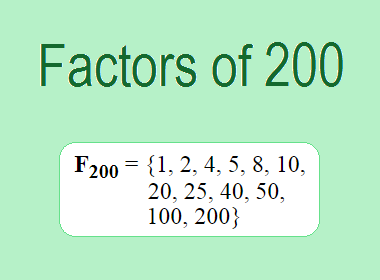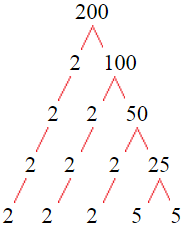# Factors of 200The factors of 200 are 1, 2, 4, 5, 8, 10, 20, 25, 40, 50, 100, and 200 i.e. F200 = {1, 2, 4, 5, 8, 10, 20, 25, 40, 50, 100, 200}. The factors of 200 are all the numbers that can divide 200 without leaving a remainder.

We can check if these numbers are factors of 200 by dividing 200 by each of them. If the result is a whole number, then the number is a factor of 200. Let's do this for each of the numbers listed above:

·        1 is a factor of 200 because 200 divided by 1 is 200.

·        2 is a factor of 200 because 200 divided by 2 is 100.

·        4 is a factor of 200 because 200 divided by 4 is 50.

·        5 is a factor of 200 because 200 divided by 5 is 40.

·        8 is a factor of 200 because 200 divided by 8 is 25.

·        10 is a factor of 200 because 200 divided by 10 is 20.

·        20 is a factor of 200 because 200 divided by 20 is 10.

·        25 is a factor of 200 because 200 divided by 25 is 8.

·        40 is a factor of 200 because 200 divided by 40 is 5.

·        50 is a factor of 200 because 200 divided by 50 is 4.

·        100 is a factor of 200 because 200 divided by 100 is 2.

·        200 is a factor of 200 because 200 divided by 200 is 1.

## How to Find Factors of 200?

1 and the number itself are the factors of every number. So, 1 and 200 are two factors of 200. To find the other factors of 200, we can start by dividing 200 by the numbers between 1 and 200. If we divide 200 by 2, we get a remainder of 0. Therefore, 2 is a factor of 200. If we divide 200 by 3, we get a remainder of 2. Therefore, 3 is not a factor of 200.

Next, we can check if 4 is a factor of 200. If we divide 200 by 4, we get a remainder of 0. Therefore, 4 is also a factor of 200. We can continue this process for all the possible factors of 200.

Through this process, we can find that the factors of 200 are 1, 2, 4, 5, 8, 10, 20, 25, 40, 50, 100, and 200. These are the only numbers that can divide 200 without leaving a remainder.

********************

********************

## Properties of the Factors of 200

The factors of 200 have some interesting properties. One of the properties is that the sum of the factors of 200 is equal to 465. We can see this by adding all the factors of 200 together:

1 + 2 + 4 + 5 + 8 + 10 + 20 + 25 + 40 + 50 + 100 + 200 = 465

Another property of the factors of 200 is that the prime factors of 200 are 2, and 5 only.

## Applications of the Factors of 200

The factors of 200 have several applications in mathematics. One of the applications is in finding the highest common factor (HCF) of two or more numbers. The HCF is the largest factor that two or more numbers have in common. For example, to find the HCF of 200 and 150, we need to find the factors of both numbers and identify the largest factor they have in common. The factors of 200 are 1, 2, 4, 5, 8, 10, 20, 25, 40, 50, 100, and 200. The factors of 150 are 1, 2, 3, 5, 6, 10, 15, 25, 30, 50, 75, and 150. The largest factor that they have in common is 50. Therefore, the HCF of 200 and 150 is 50.

Another application of the factors of 200 is in prime factorization. Prime factorization is the process of expressing a number as the product of its prime factors. The prime factors of 200 are 2, and 5 since these are the only prime numbers that can divide 200 without leaving a remainder. Therefore, we can express 200 as:

200 = 2 × 2 × 2 × 5 × 5

We can do prime factorization by division and factor tree method also. Here is the prime factorization of 200 by division method,200 = 2 × 2 × 2 × 5 × 5

Here is the prime factorization of 200 by the factor tree method,200 = 2 × 2 × 2 × 5 × 5

## Conclusion

The factors of 200 are the numbers that can divide 200 without leaving a remainder. The factors of 200 are 1, 2, 4, 5, 8, 10, 20, 25, 40, 50, 100, and 200. The factors of 200 have some interesting properties, such as having a sum of 465. The factors of 200 have several applications in mathematics, such as finding the highest common factor and prime factorization.